Work-Energy Equation combines Kinetic Energy and Potential Energy of a mechanical objectto formulate the equation as,

T1 + Vg1 + Ve1 + U1– 2 = T2 + Vg2 + Ve2

Or,

U1– 2 =  (T + Vg + Ve) = E

Where,

E = Total Mechanical Energy

Consider the value of E is constant, in such case the value of change in E will be zero such that the change in work done becomes equal to zero.

i.e.,

U1– 2 = 0

Note:

The above equation can also be defined for law of conversation of dynamic energy as the value of E is constant.

Example 6.1:

An inclined block of steel is making an angle of 300 with the horizontal as shown in figure below. The weight of the steel block is 8000 N. If an external force is applied to pull the block up to 500mm on the smooth plane, then calculate the work done.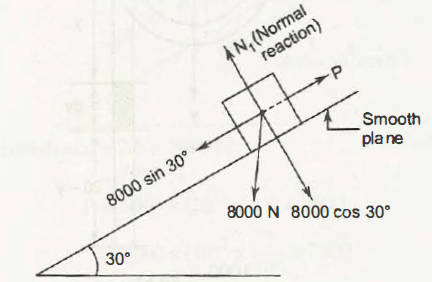Solution:

Given Data:

Component of Normal Reaction, N = 8000 cos300

Component of Force, P = 8000 sin300

= 8000 x ½                            (sin300 = ½)

= 4000

Hence,

Work done      =          P x 500/ 1000

=          4000/ 2

=          2000 J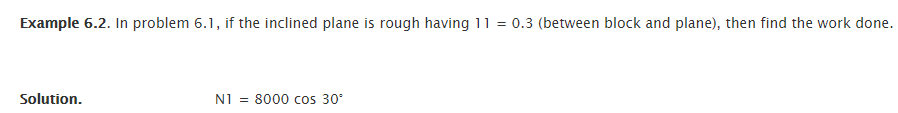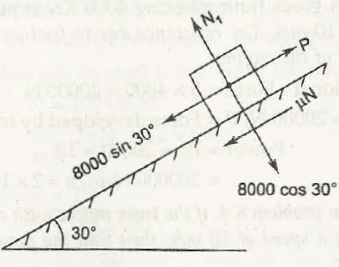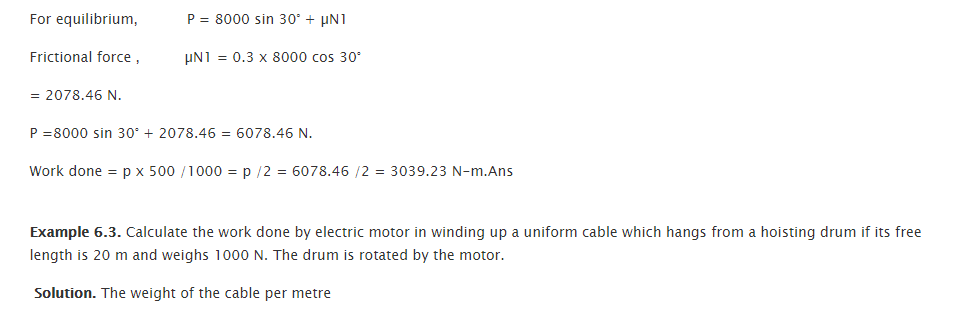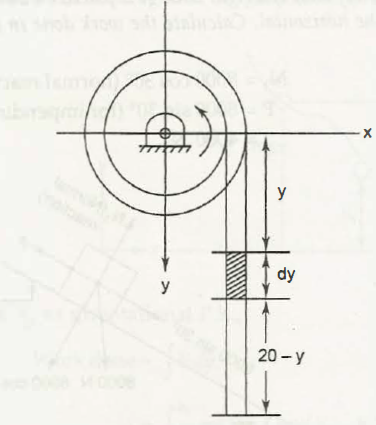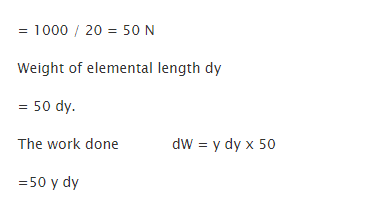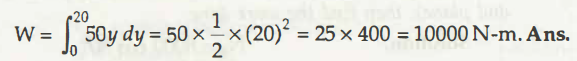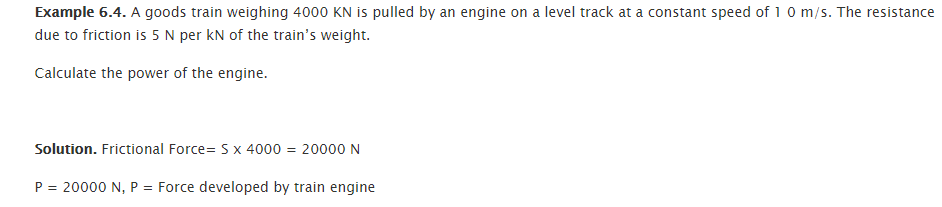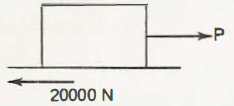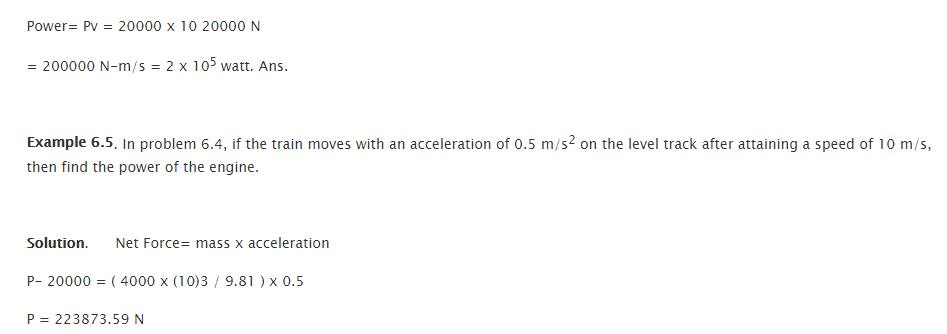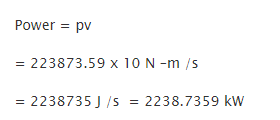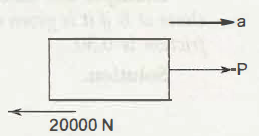Links of Next Mechanical Engineering Topics:-### Customer Reviews

My Homework Help
Rated 5.0 out of 5 based on 510 customer reviews at